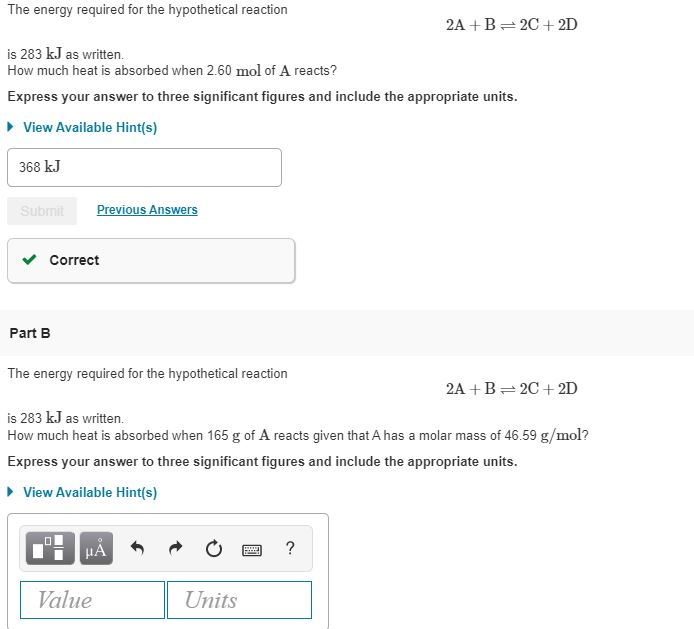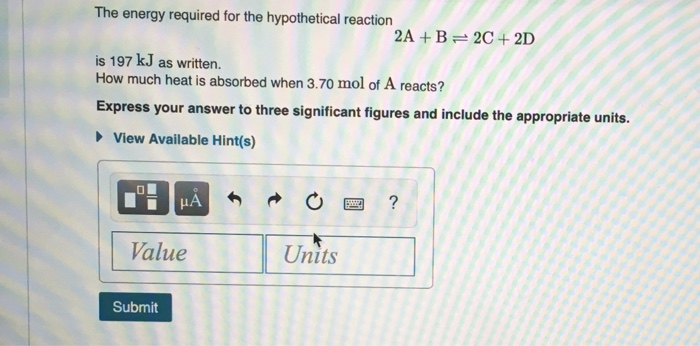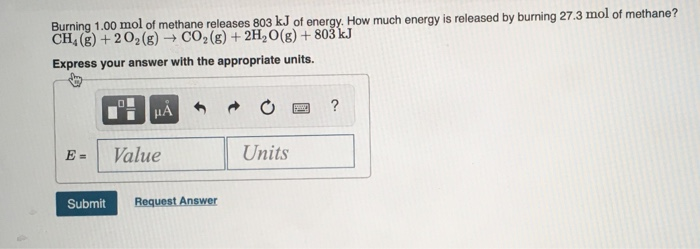# The decomposition of NI3 to form N2 and I2 releases −290.0 kJ of energy. The reaction...

The decomposition of NI3 to form N2 and I2 releases −290.0 kJ of energy. The reaction can be represented as 2NI3(s)→N2(g)+3I2(g), ΔHrxn=−290.0 kJ Find the change in enthaply when 12.0 g of NI3 decomposes. Express your answer to three significant figures and include the appropriate units.

Step 1: Explanation

When enthalpy is positive and delta H is greater than zero, this means that a system absorbed heat. This is called an endothermic reaction.

When enthalpy is negative and delta H is less than zero, this means that a system released heat. This is called an exothermic reaction

Step 2: Calculate the moles from mass

We know, moles = mass / molar mass

molar mass of NI3 = 394.719 g/mol

moles of NI3 = 12 g / 394.719 g/mol = 0.0304 mol

Step 2: Calculation of change in enthalpy due to heat released

Write the equation

2 NI3(s) --------->N2(g) + 3 I2(g); ΔH= -290 kJ

For 2 moles of NI3 enthalpy changed = -290 kJ

so, for 0.0304 mol of NI3 enthalpy change will be = ( -290 kJ / 2 mol ) × 0.0304 mol = -4.41 kJ

#### Earn Coin

Coins can be redeemed for fabulous gifts.

Similar Homework Help Questions
• ### Titanium reacts with iodine to form titanium(III) iodide, emitting heat. 2Ti(s)+3I2(g)→2TiI3(s)ΔHorxn = -839 kJ Determine the...

Titanium reacts with iodine to form titanium(III) iodide, emitting heat. 2Ti(s)+3I2(g)→2TiI3(s)ΔHorxn = -839 kJ Determine the masses of titanium and iodine that react if 1.40×103 kJ of heat is emitted by the reaction. Express your answer to three significant figures and include the appropriate units

• ### Consider the reaction between nitrogen and oxygen gas to form dinitrogen monoxide: 2N2(g)+O2(g)→2N2O(g),ΔHrxn=+163.2kJ Part A Calculate...

Consider the reaction between nitrogen and oxygen gas to form dinitrogen monoxide: 2N2(g)+O2(g)→2N2O(g),ΔHrxn=+163.2kJ Part A Calculate the entropy change in the surroundings associated with this reaction occurring at 25∘C. Express the entropy change to three significant figures and include the appropriate units.

• ### Chemical energy stored in the reactants is released as heat when the reaction is exothermic. For...

Chemical energy stored in the reactants is released as heat when the reaction is exothermic. For an exothermic reaction, the enthalpy change for a reaction is negative. Enthalpy, represented as H, is the heat content of a system. As it is a state function, it depends on the state of the system; hence, it is calculated in the form of change in heat content. Heat change, or enthalpy change, is represented as ΔH; ΔH is a measure of the heat...

• ### The energy required for the hypothetical reaction 2A+B=2C+2D is 283 kJ as written. How much heat...The energy required for the hypothetical reaction 2A+B=2C+2D is 283 kJ as written. How much heat is absorbed when 2.60 mol of A reacts? Express your answer to three significant figures and include the appropriate units. View Available Hint(s) 368 kJ Submit Previous Answers Correct Part B The energy required for the hypothetical reaction 2A+B=2C+2D is 283 kJ as written. How much heat is absorbed when 165 g of A reacts given that A has a molar mass of 46.59...

• ### The energy required for the hypothetical reaction 2A+ B 2C+ 2D is 197 kJ as written....The energy required for the hypothetical reaction 2A+ B 2C+ 2D is 197 kJ as written. How much heat is absorbed when 3.70 mol of A reacts? Express your answer to three significant figures and include the appropriate units. View Available Hint(s) ? Units Value Submit

• ### Burning 1.00 mol of methane releases 803 kJ of energy. How much energy is released by...Burning 1.00 mol of methane releases 803 kJ of energy. How much energy is released by burning 27.3 mol of methane? CH, (g) +202(g) + CO2(g) + 2H2O(g) + 803 kJ Express your answer with the appropriate units. E- Value Units Submit Request Answer

• ### The reaction N2(g)+O2(g)⇌2NO(g)  is carried out at a temperature at which Kc = 0.050. The reaction mixture...

The reaction N2(g)+O2(g)⇌2NO(g)  is carried out at a temperature at which Kc = 0.050. The reaction mixture starts with only the product, [NO] = 0.0300 M , and no reactants. Part A Find the equilibrium concentrations of N2 at equilibrium. Express your answer to two significant figures and include the appropriate units. [N2] = Part B Find the equilibrium concentrations of O2 at equilibrium. Part C Find the equilibrium concentrations of NO at equilibrium. Express your answer to two significant figures...

• ### For the reaction H2(g)+I2(g)⇌2HI(g), Kc= 55.3 at 700 K. In a 2.00-L flask containing an equilibrium...

For the reaction H2(g)+I2(g)⇌2HI(g), Kc= 55.3 at 700 K. In a 2.00-L flask containing an equilibrium mixture of the three gases, there are 0.053 g H2 and 4.38 g I2. What is the mass of HI in the flask? Express your answer to two significant figures and include the appropriate units.

• ### Burning 1.00 mol of methane releases 803 kJ of energy. How much energy is released by...

Burning 1.00 mol of methane releases 803 kJ of energy. How much energy is released by burning 27.7 mol of methane? CH4(g)+2O2(g)→CO2(g)+2H2O(g)+803kJ Express your answer with the appropriate units.

• ### Can you please help me calculate part E??? Part A Calculate the bond energy per mole...

Can you please help me calculate part E??? Part A Calculate the bond energy per mole for breaking all the bonds in methane, CH4. Express your answer to four significant figures and include the appropriate units. ΔHCH4 = 1656 kJmol Correct In CH4, the energy required to break one C−H bond is 414 kJ/mol. Since there are four C−H bonds in CH4, the energy ΔHCH4 for breaking all the bonds is calculated as ΔHCH4 = 4×bond energy of C−H bond...